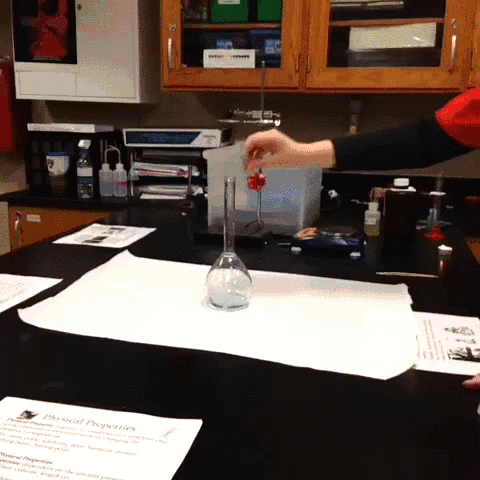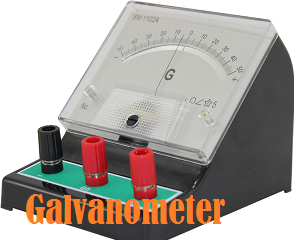## Catalytic Decomposition of H2O2 – Elephant’s Toothpaste Experiment

Elephant's toothpaste is a foamy substance formed as a result of rapid decomposition of hydrogen peroxide. Elephant’s toothpaste reaction experiment is generally used for classroom demonstrations as it requires fewer ingredients and makes a "volcano of foam".

#### Description:

H2O2 is decomposed by Iodide Ion. Iodide ion is used as a catalyst and these iodide ions come from KI (Potassium Iodide) or NaI (Sodium Iodide). This decomposition reaction liberates water, oxygen and heat.

#### Materials:

30% H2O2 (Hydrogen Peroxide)
KI (Potassium Iodide) or NaI (Sodium Iodide).
Food Color for fun
Liquid Dish Detergent

#### Procedure:

1.  Pour 50 mL of 30% H2O2 (Hydrogen Peroxide) in 1 L graduated cylinder.
2.  Then, add 3-4 drops of food color in 1 L graduated cylinder to make colored H2O2 solution.
3.  Then, start adding liquid dish detergent or soap from the side of 1 L graduated cylinder to make a small layer of liquid dish detergent or soap over colored H2O2 solution.
4.  With the help of small beaker add KI or NaI in the cylinder containing H2O2 solution. As a result of reaction, oxygen gas generated and this gas creates large amount of colored foam. This foam comes out of graduated cylinder.

#### Discussion:

Decomposition of H2O2 (Hydrogen Peroxide) yields O2 (oxygen) and H2O (water). Reaction is catalyzed by I- (Iodide Ion); These Iodide Ion comes from KI or NaI

#### Reaction-

H2O2 (Aq) + I-1 (Aq) ---> H2O (liquid) + IO- (Aq)
IO- (Aq) + H2O2 (Aq) ---> H2O (liquid) +O2 (g) + I- (Aq)

#### Overall Reaction-

2H2O2 (Aq) ---> 2H2O (liquid) +O2 (g)

#### Safety:

Wear proper protective equipment likes hand-gloves and safety goggles when preparing and demonstrating this experiment. Remember concentrated H2O2 (Hydrogen Peroxide) can cause burns.

## Chemical Reaction Quiz

Science Quiz by www.ChemistryNotesInfo.comSource www.reddit.com
This Chemical Reaction Quiz part16 is based on chemical reactions.

#### 1. Chemical reaction always gives-

Reactants
A change of state
A different substance
Water
All of the above

#### 2. From given below, 4-balanced chemical equations, which is example of combustion reaction-

2Mg + O2 → 2MgO
2N2 + 3H2 → 2NH3
CaO + H2O → Ca(OH)2
2Na + Cl2 → 2NaCl
2CH4 + 4O2 → 2CO2 + 4H2O

#### 3. To balance a chemical equation, you should need to change-

Chemical Formulas
Direction of the arrow
Coefficients
Subscripts
Superscripts

#### 4. In chemistry, if you try to balance a chemical equation by changing its subscripts, than you change the-

Atomic number
Atomic Mass
Mass of the reactants
Number of molecules of product
Identity of the compound

#### 5. From given below, 4-balanced chemical equations, which is example of double displacement reaction-

NH4Cl → NH3 + HCl
Cd(NO3)2 + Na2S → CdS + 2NaNO3
2Mg + O2 → 2MgO
2H2O2 → 2H2O + O2
2N2 + 3H2 → 2NH3

#### 6. Chemical Reaction, KClO3 → KCl + O2 is a-

Synthesis Reaction
Decomposition Reaction
Combustion Reaction
Single Displacement Reaction
Double Displacement Reaction

Lavoisier
Galileo
Newton
Archimedes
Pasteur

#### 8. Combination reactions (also known as Synthesis Reaction) always-

Involve an element and an ionic compound
Require oxygen gas
Use only one reactant
Form only one product
form only two product

#### 9. In a chemical reaction, the arrow means-

A reaction is happening
The equation is balanced
The products flow into reactants
The two sides are equal
All of the above

#### 10. In a decomposition reaction-

Energy in the form of light or heat is often released
Reactants are commonly two ionic compounds in the aqueous solution
one of the reactant is generally water
Form only one product
Reactants in the chemical reaction are usually a metal and a nonmetal## Chemical Reactions Quiz

Science Quiz by www.ChemistryNotesInfo.com
This Chemical Reactions Quiz part15 is based on chemical reaction.

#### 1. Chemical Reaction, 2H₂O → 2H₂ + O₂ is a-

Synthesis Reaction
Decomposition Reaction
Combustion Reaction
Single Displacement Reaction
Double Displacement Reaction

#### 2. Chemical Reaction, 8Fe + S₈ → 8FeS is a-

Synthesis Reaction
Decomposition Reaction
Combustion Reaction
Single Displacement Reaction
Double Displacement Reaction

#### 3. Chemical Reaction, Zn + H₂SO₄ → ZnSO₄ + H₂ is a-

Synthesis Reaction
Decomposition Reaction
Combustion Reaction
Single Displacement Reaction
Double Displacement Reaction

#### 4. Chemical Reaction, AgNO₃ + NaCl → AgCl + NaNO₃ is a-

Synthesis Reaction
Decomposition Reaction
Combustion Reaction
Single Displacement Reaction
Double Displacement Reaction

#### 5. Chemical Reaction, CH₄ + 2O₂ → CO₂ + 2H₂O is a-

Synthesis Reaction
Decomposition Reaction
Combustion Reaction
Single Displacement Reaction
Double Displacement Reaction

#### 6. Chemical Reaction, 2CO + O₂ → 2CO₂ is a-

Synthesis Reaction
Decomposition Reaction
Combustion Reaction
Single Displacement Reaction
Double Displacement Reaction

#### 7. Chemical Reaction, Ca(OH)₂ + H₂SO₄ → CaSO₄ + 2H₂O is a-

Synthesis Reaction
Decomposition Reaction
Combustion Reaction
Single Displacement Reaction
Double Displacement Reaction

#### 8. Chemical Reaction, Pb + O₂ → PbO₂ is a-

Synthesis Reaction
Decomposition Reaction
Combustion Reaction
Single Displacement Reaction
Double Displacement Reaction

#### 9. Chemical Reaction, 2Fe + 6NaBr → 2FeBr₃ + 6Na is a-

Synthesis Reaction
Decomposition Reaction
Combustion Reaction
Single Displacement Reaction
Double Displacement Reaction

#### 10. Chemical Reaction, 2H₂ + O₂ → 2H₂O is a-

Synthesis Reaction
Decomposition Reaction
Combustion Reaction
Single Displacement Reaction
Double Displacement Reaction

## Science Quiz on Measuring Devices

Science Quiz by www.ChemistryNotesInfo.com
This Science Quiz part14 is based on different measuring devices or measuring instruments used in the field of Science.

#### 1. Distance traveled, displacement is measured by:

Taxmeter
Taximeter
Distancemeter
Displacementmeter

Tacheometer
Tintometer
Tensiometer
Tachometer

Thermometer
Thermusmeter
Hygrometer
Hydrometer

#### 4. Ultraviolet light is measured by:

EW Meter
Electromagnetic Wave Meter
UV Meter
VU Meter

Pressure Meter
Voltmeter
Viscometer
Vacuum Gauge

#### 6. Viscosity of a fluid is measured by:

Viscosity Meter
Viscometer
Ostwald Viscometer Principle
Viscosity Measurement Devices

#### 7. Electric potential, voltage is measured by:

Voltmeter
Digital DC Voltmeter
Analog Panel Voltmeter
Ammeter

Ammeter
Wattmeter
Voltmeter
Voltage Meter

Wind Vane
Weather Vane
Wind Vane Scale
Wind Vane Meter

#### 10. Fermentation is measured by:

fermentometer
Fermometer
Fermentation Meter
Zymometer

## Science Quiz on Measuring Devices

Science Quiz by www.ChemistryNotesInfo.com
This Science Quiz part13 is based on different measuring devices or measuring instruments used in the field of Science.

#### 1. Color is measured by:

Tintometer
Color Meter
Color Identification Devices
Color Measuring Instrument

#### 2. Speed, velocity of the vehicle is measured by:

Speed Detector
Speed Tester
Speedometer
None of the above

#### 3. Lung capacity is measured by:

ECG Machine
Sphygmomanometers
Stethoscopes
Spirometer

#### 4. Blood pressure is measured by:

Digital Blood Pressure Monitor
Clinical Thermometers
Glucometer
Sphygmomanometer

Ruler
Spherometer
Diameter
Scale

Kilometer
Milimeter
Meter

Science Quiz
Strainmetere
Refractometer
Seismicmeter

SWR Meter
Wave Ratio Meter
Electrometer

#### 9. Distance is measured by:

Distance Meter
Laser Distance
Distance Measurement Device
Tacheometer

#### 10. Revolutions per minute (RPM), speed of aeroplanes, rate of blood flow is measured by:

Tachometer
Tacheometer
Digital RPM Meter
RPM Meter## Science Quiz on Measuring Devices

Chemistry GK by www.ChemistryNotesInfo.com
This Science Quiz part12 is based on different measuring devices or measuring instruments used in the field of Science.

#### 1. Intensity of the light as a function of the wavelength is measured by:

Spectrometer
Spectrophotometer
Spectroscopy
Color Spectrophotometer

#### 2. Properties of light is measured by:

Spectrometer
Spectrophotometer
Spectroscopy
Color Spectrophotometer

#### 3. Seismic waves (during earthquakes) is measured by:

Seismic Waves Meter
Surface Buoys
Seismometer
Underwater Sensors

Saccharometer
Sugar Meter
Glucometer
Sugar Tester

Ruler
Insurance
Classes
Degree

Watt-hour meter
Q meter
Rheometer
Rotameter

Hygrometer
RF/Microwave
Refractometer
Hydrometer

Audiometer
Electrometer

#### 9. Thickness of the deposited thin films is measured by:

QC Lab Microbalance
Standard Films Microbalance
Quartz Microbalance
Quartz Crystal Microbalance

#### 10. High temperatures is measured by:

Thermometer
Pyrometer
Hygrometer
Pyranometer## Science Quiz

Chemistry GK by www.ChemistryNotesInfo.com
This Science Quiz part11 is based on different measuring devices or measuring instruments used in the field of Science.

Osmosisindicator
pH Meter
Osmometer
Rotameter

Pyrometer
pH Meter
Ohmmeter
Berometer

Photometer
Light Meter

Photometer
Light Meter
Polarimeter

Potentiometer
Orchidometer
Ohmmeter
Ammeter

#### 6. Surface roughness is measured by:

Mass Spectrometer
Mass Flow Meter
Nephelometer
Profilometer

Hydrometer
Protractor
Scale
Inkometer

Hydrometer
Glucometer
Psychrometer
Electrometer

Potentiometer
Pycnometer
Psychrometer
Interferometer

#### 10. Solar radiation is measured by:

Thermometer
Rotameter
Rular
Pyranometer## Science General Knowledge

Chemistry GK by www.ChemistryNotesInfo.com
This chemistry GK part10 is based on different measuring devices or measuring instruments used in chemistry and science.

### Q1.Measurement of force is measured by• Mass Cell
• Weight Cell
Ans of Chemistry GK MCQ Question Q1: Load Cell

### Q2.Intensity of light is measured by

• Lux Meter
• Light Meter
• Photometer
• Disdrometer
Ans of Chemistry GK MCQ Question Q2: Lux Meter

### Q3.The mass flow rate of a fluid traveling through the tube is measured by

• Fluid Flow Meter
• Volume Flow Meter
• Mass flow meter
• Volume Flow Gauge
Ans of Chemistry GK MCQ Question Q3: Mass flow meter

### Q4. ______ is used in measurement of strength of magnetic fields

• Polometer
• Bolometer
• Magnetometer
Ans of Chemistry GK MCQ Question Q4: Magnetometer

### Q5.The masses of ions, used to identify chemical substance or chemical substances through their mass spectra is measured by

• Optical Emission Spectrometer
• Mass Spectrometer
• Modular Spectrometer
• UV-Visible Spectrometer
Ans of Chemistry GK MCQ Question Q5: Mass Spectrometer

### Q6.____ device is used to measure electrical potential, resistance, and current

• Multimeter
• Potentiometer
• Digital Multimeter
• Analog Multimeter
Ans of Chemistry GK MCQ Question Q6: Multimeter

### Q7. _______ is use to measure atmospheric pressure

• Atmosphere Barometer
• Arab Barometer
• Mercury Barometer
• Air Barometer
Ans of Chemistry GK MCQ Question Q7: Mercury Barometer

### Q8. Device use to measure the speed and direction of clouds

• Nephoscope
• Nephoscoper
• Mirror Nephoscope
• Nefoscope
Ans of Chemistry GK MCQ Question Q8: Nephoscope

### Q9. ______ is use to measure electrical resistance

• Chemical Resistance Meter
• Shunt
• Resistance Meter
• Ohm Meter
Ans of Chemistry GK MCQ Question Q9: Ohmmeter

### Q10.Oscillations is measured by

Ans of Chemistry GK MCQ Question Q10: Oscilloscope

We publish some chemistry general knowledge question-answer (Chemistry MCQs) to increase your chemical science GK.<= Chemistry GK Part9

## Chemistry MCQ

Chemistry GK by www.ChemistryNotesInfo.com
This chemistry GK part9 is based on different measuring devices or measuring instruments used in chemistry and science.

### Q1.Fuel level is measured by

• Speedometer
• Fuel Scale
• Fuel Meter
• Fuel Gauge
Ans of Chemistry GK MCQ Question Q1: Fuel Gauge

### Q2. Electricity is measured by• Ammeter
• Galvanometer
• Amplifier
• Currentmeter
Ans of Chemistry GK MCQ Question Q2: Galvanometer

### Q3.Volume and density of solids is measured by

• Dilatometer
• Caliper
• Densimeter
• Gas Pycnometer
Ans of Chemistry GK MCQ Question Q3: Gas Pycnometer

### Q4. ______ is used in measurement of blood glucose (diabetes)

• Glucometer
• Blood Glucose Monitor or Glucose Tester
• Glucose Meter
• Glucometer or Glucose Meter
Ans of Chemistry GK MCQ Question Q4: Glucometer and Glucose Meter

### Q5.Specific gravity of liquids (density of liquids) is measured by

• Fathometer
• Thermometer
• Hygrometer
• Hydrometer
Ans of Chemistry GK MCQ Question Q5: Hydrometer

### Q6.____ device is used to measure humidity

• Dynamometer
• Thermometer
• Hygrometer
• Hydrometer
Ans of Chemistry GK MCQ Question Q6: Hygrometer

### Q7. _______ is use to measure wave interference

• Actinometer
• Elaeometer
• Interferometer
Ans of Chemistry GK MCQ Question Q7: Interferometer

### Q8. Device use to measure composition of gases

• Heliometer
• Inkometer
• Katharometer
• Lux Meter
Ans of Chemistry GK MCQ Question Q8: Katharometer

### Q9. ______ is use to measure specific gravity of milk

• Gasometer
• Lactometer
• Milk Meter
• Chemical Tester
Ans of Chemistry GK MCQ Question Q9: Lactometer

### Q10.Light in photography is measured by

• Light Meter
• Ellipsometer
• Photometer
• Light detector
Ans of Chemistry GK MCQ Question Q10: Light Meter

We publish some chemistry general knowledge question-answer (Chemistry MCQs) to increase your chemical science GK.